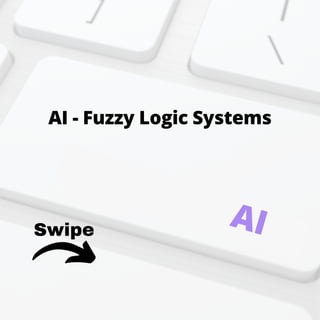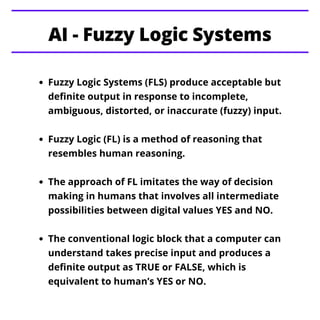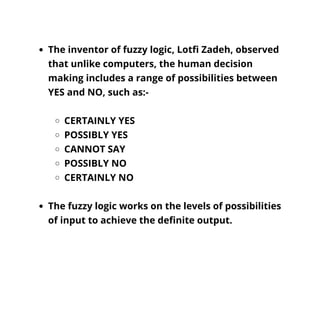Ce diaporama a bien été signalé.
Le téléchargement de votre SlideShare est en cours. ×

# AI - Fuzzy Logic Systems

Publicité
Publicité
Publicité
Publicité
Publicité
Publicité
Publicité
Publicité
Publicité
Publicité
PublicitéPublicité
Publicité
Chargement dans…3
×

## Consultez-les par la suite

1 sur 12 Publicité

# AI - Fuzzy Logic Systems

This presentation educates you about AI - Fuzzy Logic Systems and its Implementation, Why Fuzzy Logic?, Why Fuzzy Logic?, Membership Function, Example of a Fuzzy Logic System and its Algorithm.

For more topics stay tuned with Learnbay.

This presentation educates you about AI - Fuzzy Logic Systems and its Implementation, Why Fuzzy Logic?, Why Fuzzy Logic?, Membership Function, Example of a Fuzzy Logic System and its Algorithm.

For more topics stay tuned with Learnbay.

Publicité
Publicité

### AI - Fuzzy Logic Systems

1. 1. Swipe AI - Fuzzy Logic Systems AI
2. 2. Fuzzy Logic Systems (FLS) produce acceptable but definite output in response to incomplete, ambiguous, distorted, or inaccurate (fuzzy) input. Fuzzy Logic (FL) is a method of reasoning that resembles human reasoning. The approach of FL imitates the way of decision making in humans that involves all intermediate possibilities between digital values YES and NO. The conventional logic block that a computer can understand takes precise input and produces a definite output as TRUE or FALSE, which is equivalent to human’s YES or NO. AI - Fuzzy Logic Systems
3. 3. The inventor of fuzzy logic, Lotfi Zadeh, observed that unlike computers, the human decision making includes a range of possibilities between YES and NO, such as:- CERTAINLY YES POSSIBLY YES CANNOT SAY POSSIBLY NO CERTAINLY NO The fuzzy logic works on the levels of possibilities of input to achieve the definite output.
4. 4. Implementation It can be implemented in systems with various sizes and capabilities ranging from small micro- controllers to large, networked, workstation- based control systems. It can be implemented in hardware, software, or a combination of both.
5. 5. Why Fuzzy Logic? Fuzzy logic is useful for commercial and practical purposes. It can control machines and consumer products. It may not give accurate reasoning, but acceptable reasoning. Fuzzy logic helps to deal with the uncertainty in engineering.
6. 6. Fuzzy Logic Systems Architecture It has four main parts as shown:- Fuzzification Module − It transforms the system inputs, which are crisp numbers, into fuzzy sets. It splits the input signal into five steps such as:- LP - x is Large Positive MP - x is Medium Positive S - x is Small MN - x is Medium Negative LN - x is Large Negative Knowledge Base − It stores IF-THEN rules provided by experts.
7. 7. Defuzzification Module − It transforms the fuzzy set obtained by the inference engine into a crisp value. The membership functions work on fuzzy sets of variables.
8. 8. Membership Function Membership functions allow you to quantify linguistic term and represent a fuzzy set graphically. A membership function for a fuzzy set A on the universe of discourse X is defined as μA:X →[0,1]. Here, each element of X is mapped to a value between 0 and 1. It is called membership value or degree of membership. It quantifies the degree of membership of the element in X to the fuzzy set A. x axis represents the universe of discourse. y axis represents the degrees of membership in the [0, 1] interval.
9. 9. All membership functions for LP, MP, S, MN, and LN are shown as below:- The triangular membership function shapes are most common among various other membership function shapes such as trapezoidal, singleton, and Gaussian. Here, the input to 5-level fuzzifier varies from -10 volts to +10 volts. Hence the corresponding output also changes.
10. 10. Example of a Fuzzy Logic System Let us consider an air conditioning system with 5- level fuzzy logic system. This system adjusts the temperature of air conditioner by comparing the room temperature and the target temperature value.
11. 11. Algorithm Define linguistic Variables and terms (start) Construct membership functions for them. (start) Construct knowledge base of rules (start) Convert crisp data into fuzzy data sets using membership functions. (fuzzification) Evaluate rules in the rule base. (Inference Engine) Combine results from each rule. (Inference Engine) Convert output data into non-fuzzy values. (defuzzification)
12. 12. AI - Working of ANNs AI - issues and Terminology Python - Data structure Topics for next Post Stay Tuned with